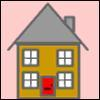#### You may also like### Odd Differences

The diagram illustrates the formula: 1 + 3 + 5 + ... + (2n - 1) = nÂ² Use the diagram to show that any odd number is the difference of two squares.### Iff### Smith and Jones

Mr Smith and Mr Jones are two maths teachers. By asking questions, the answers to which may be right or wrong, Mr Jones is able to find the number of the house Mr Smith lives in... Or not!

# Triangular Triples

##### Age 14 to 16Challenge Level

Three numbers a, b and c are a Pythagorean triple if $a^2+ b^2= c^2$. The triangular numbers are:

$\frac{1\times 2}{2}, \frac{2\times 3}{2}, \frac{3\times 4}{2}, \frac{4\times 5}{2}$

Show that 8778, 10296 and 13530 are three triangular numbers and that they form a Pythagorean triple.

[In fact, these are the ONLY known set of three triangular numbers that form a Pythagorean triple.]